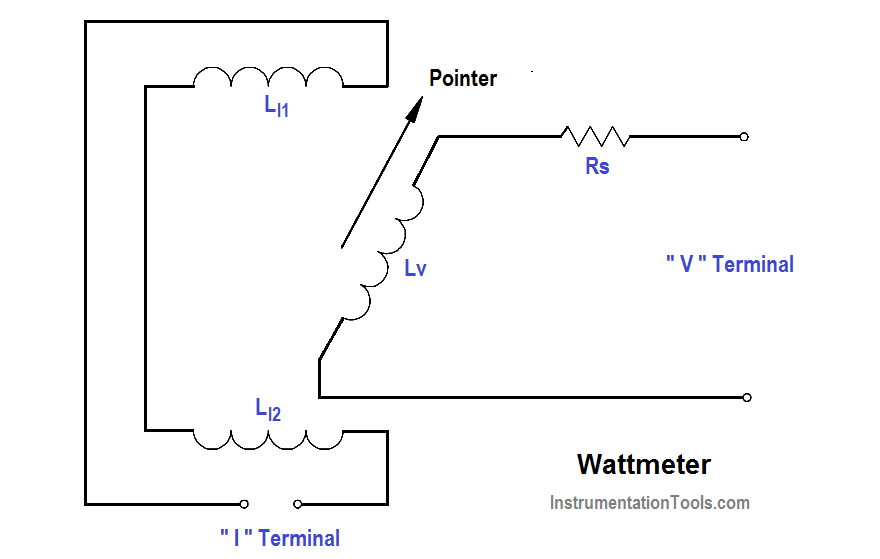# Wattmeter

The wattmeter is an instrument which measures DC power or true AC power. The wattmeter uses fixed coils to indicate current, while the movable coil indicates voltage (as shown in below Figure). Coils LI1 and LI2 are the fixed coils in series with one another and serve as an ammeter.

The two ” I ” terminals are connected in series with the load. The movable coil LV , and its multiplier resistor RS , are used as a voltmeter, with the ” V ”  terminals connected in parallel with the load. The meter deflection is proportional to the VI, which is power.Wattmeters are rated in terms of their maximum current, voltage, and power. All of these ratings must be observed to prevent damage to the meter.

The below Equation is the mathematical representation of calculating power in a DC circuit.

P = VI     or      P = I2 R

The below Equation is the mathematical representation for calculating power in an AC circuit.

P = VRMS IRMS Cos θ    or      P = I2 R